# RS Aggarwal Solutions for Class 9 Chapter 1: Number Systems Exercise 1A

## RS Aggarwal Solutions for Class 9 Maths Exercise 1A PDF

At BYJU’S, we have a set of expert faculties trying their best to provide exercise-wise solutions to students according to their level of understanding. RS Aggarwal Solutions Class 9 Chapter 1 Number Systems Exercise 1A can be used as a mode of reference by the students to improve their conceptual knowledge and understand the different ways used to solve the problems. Exercise 1A in Chapter 1 helps students to know the methods to find rational numbers between two given numbers. RS Aggarwal Solutions for Class 9 Maths Chapter 1 Number Systems Exercise 1A are provided here.

## RS Aggarwal Solutions for Class 9 Chapter 1: Number Systems Exercise 1A Download PDF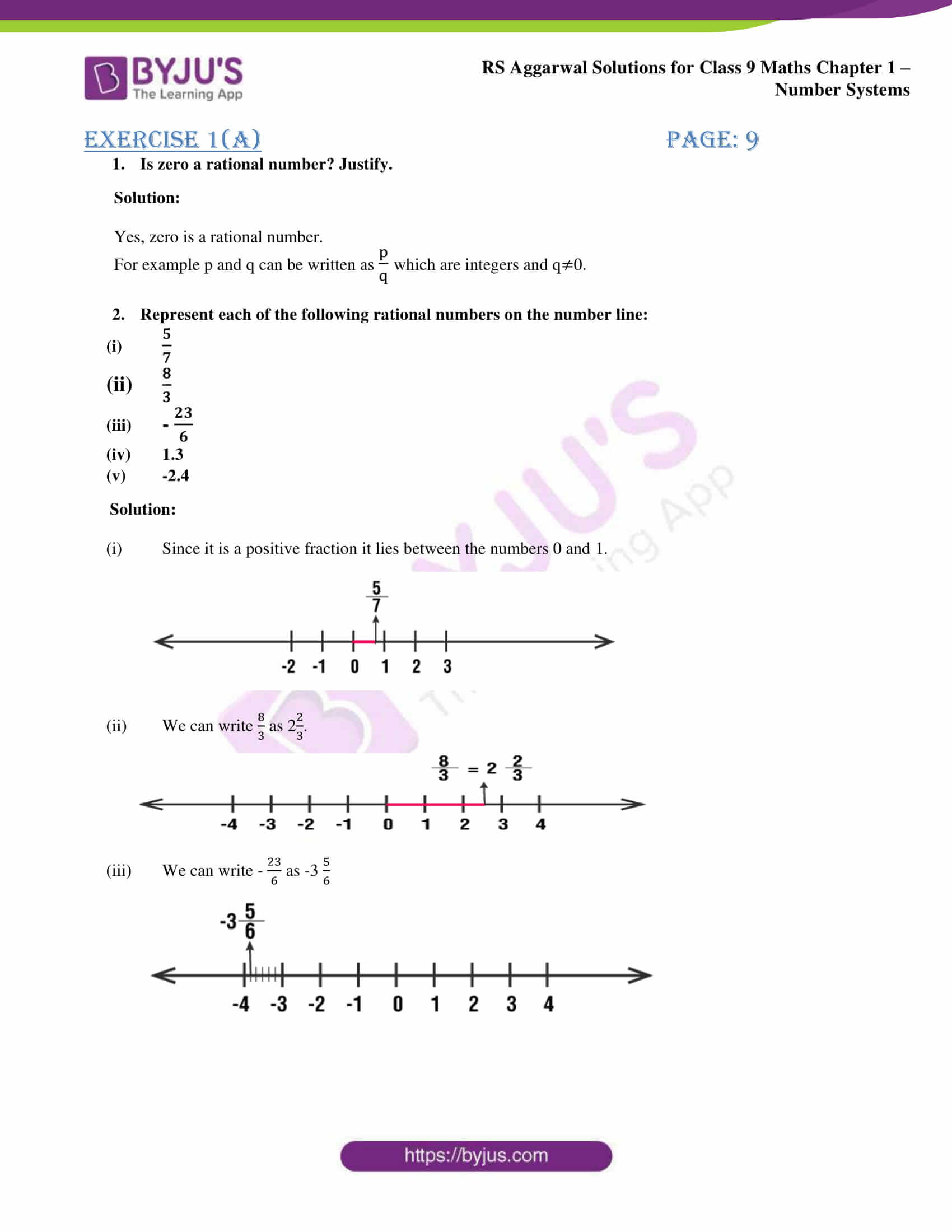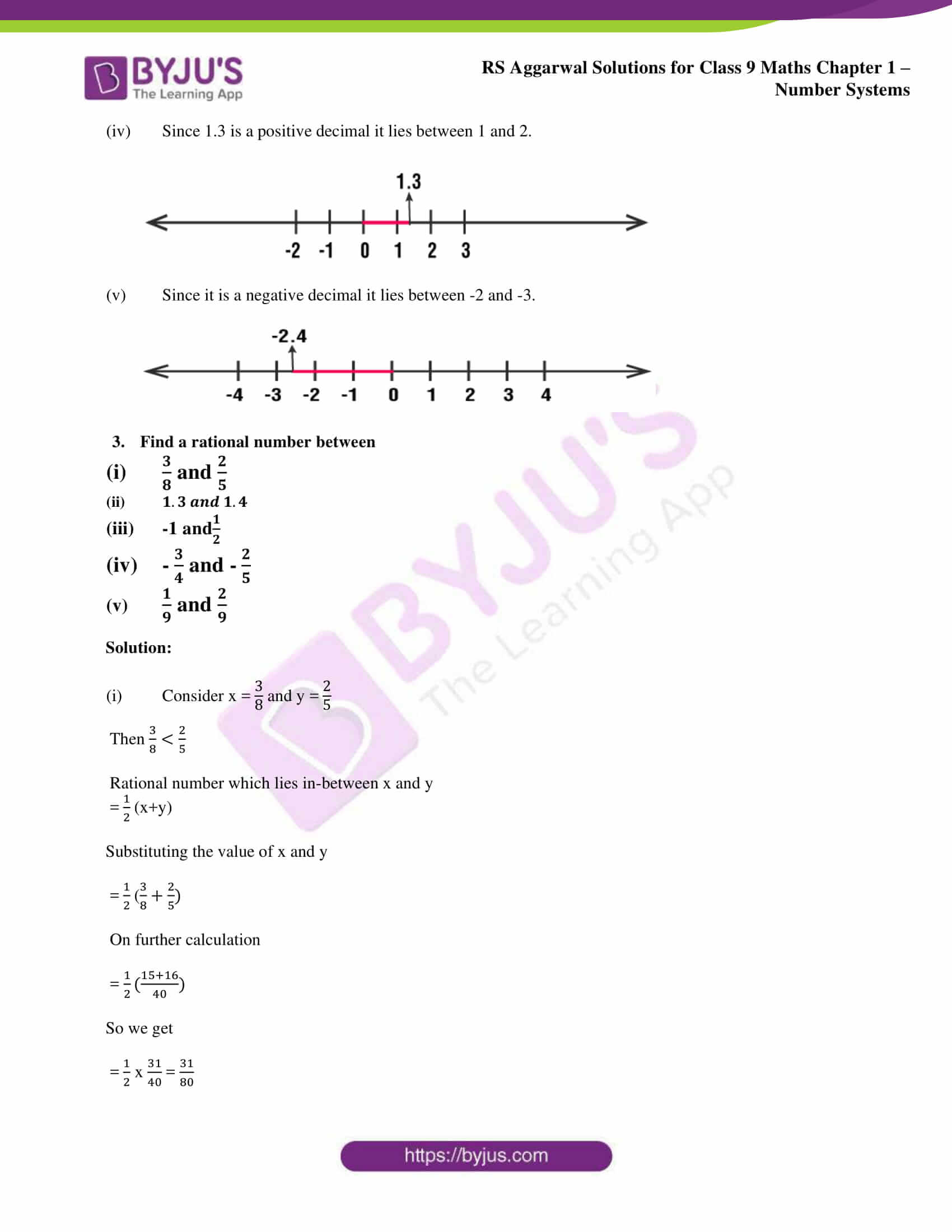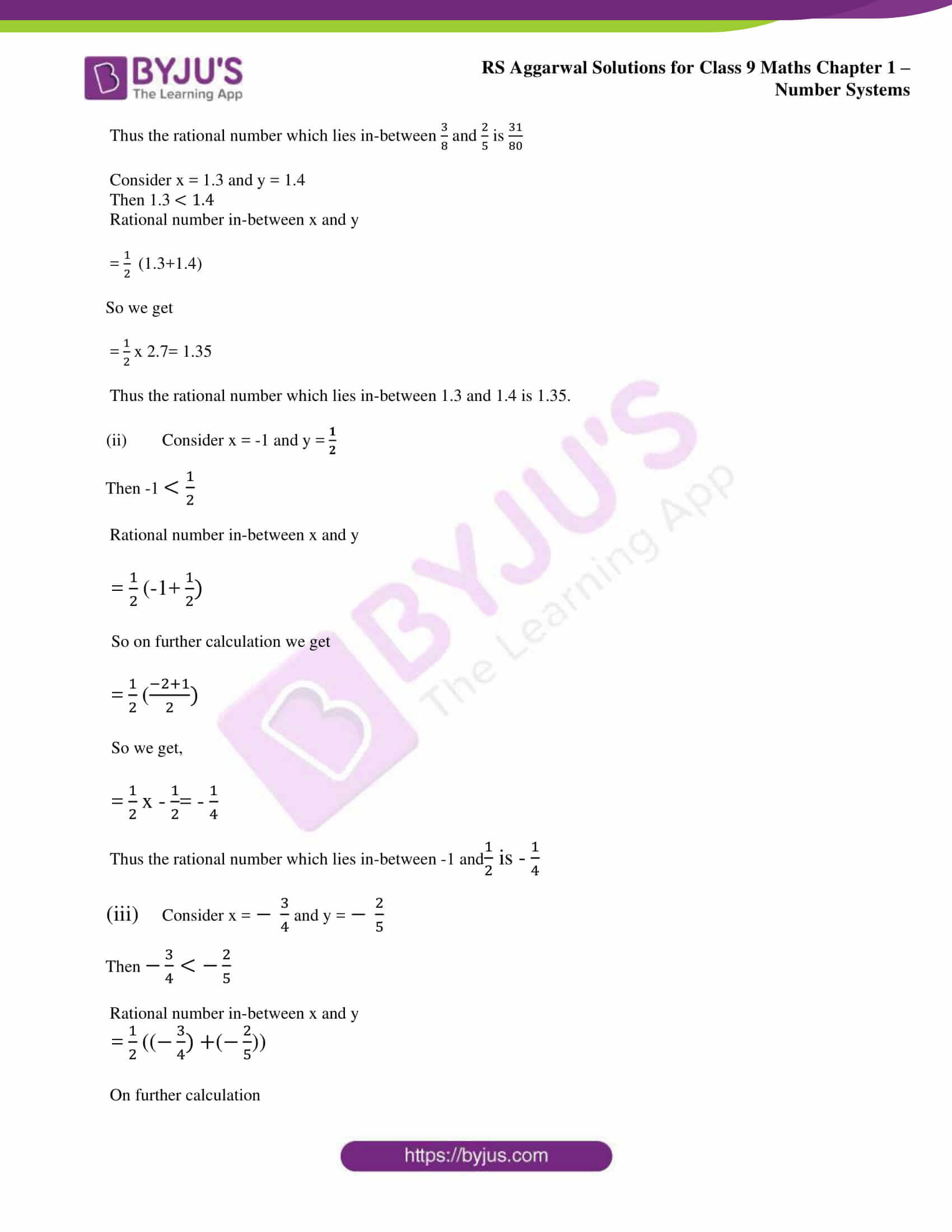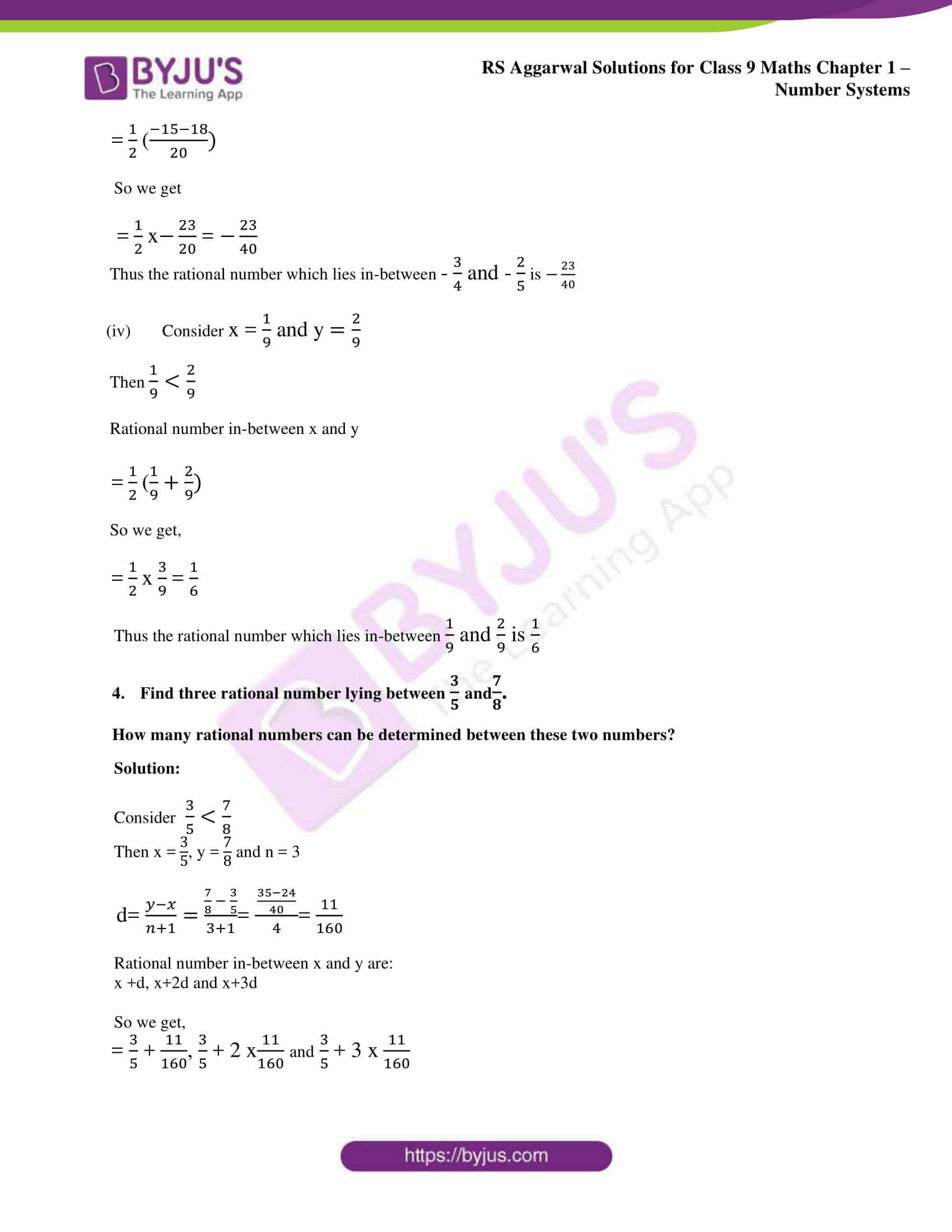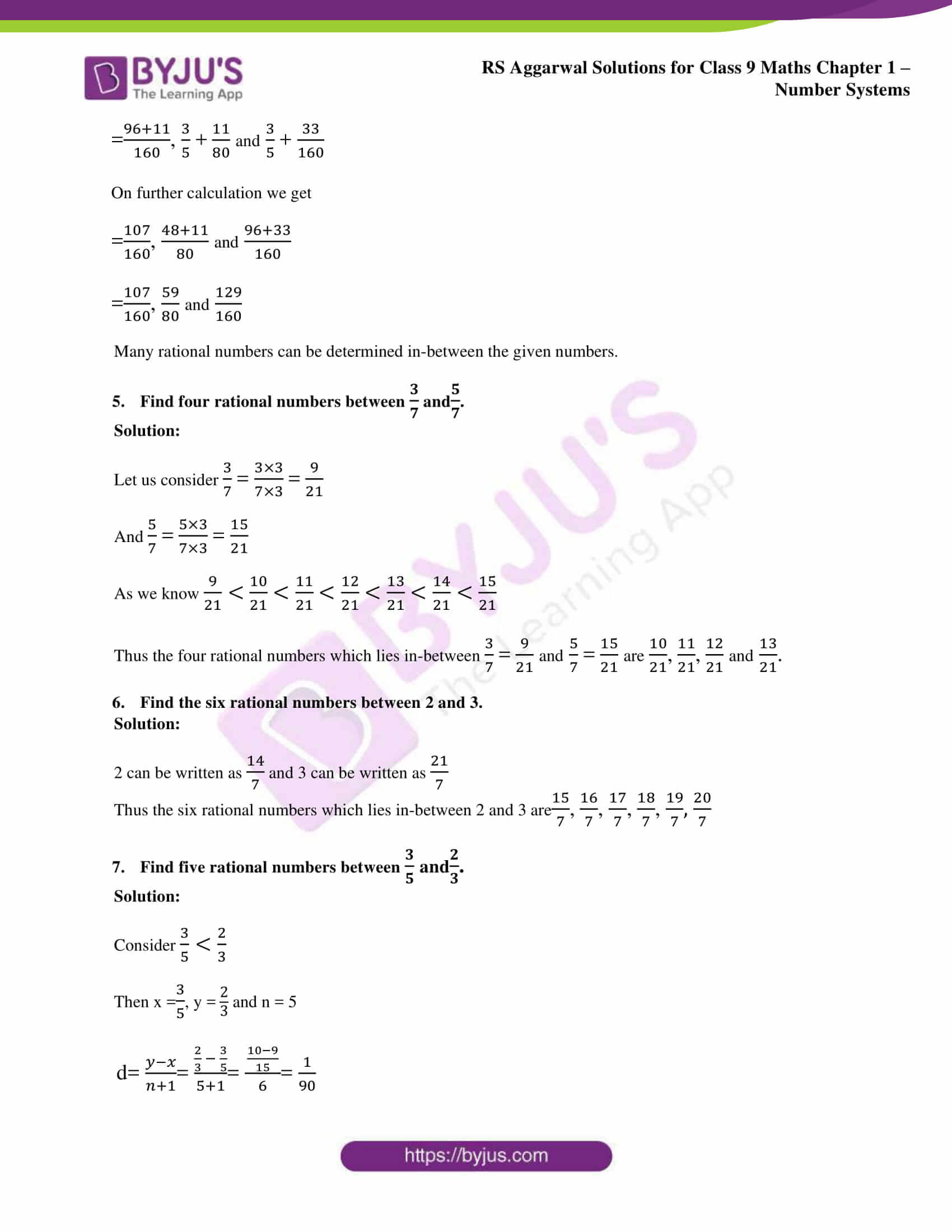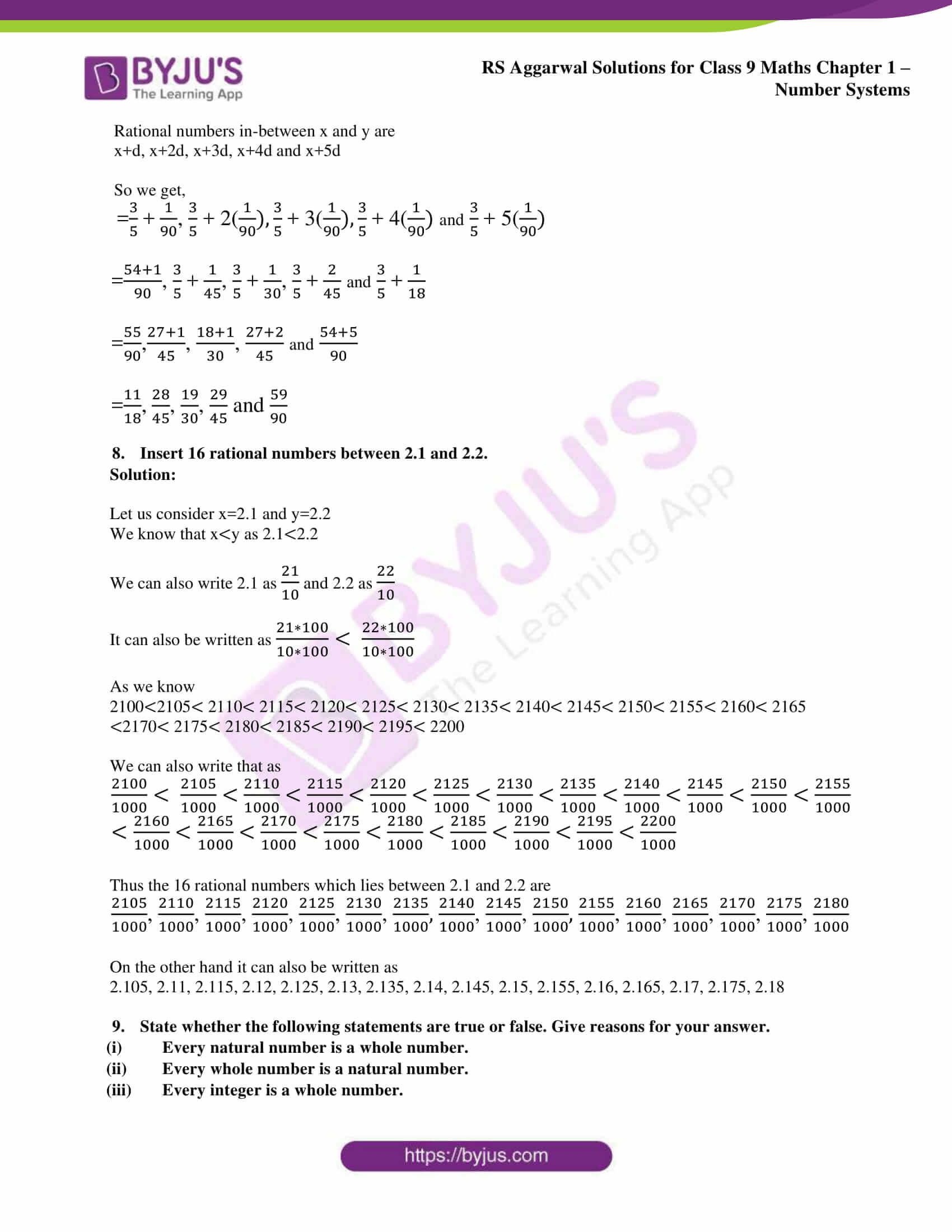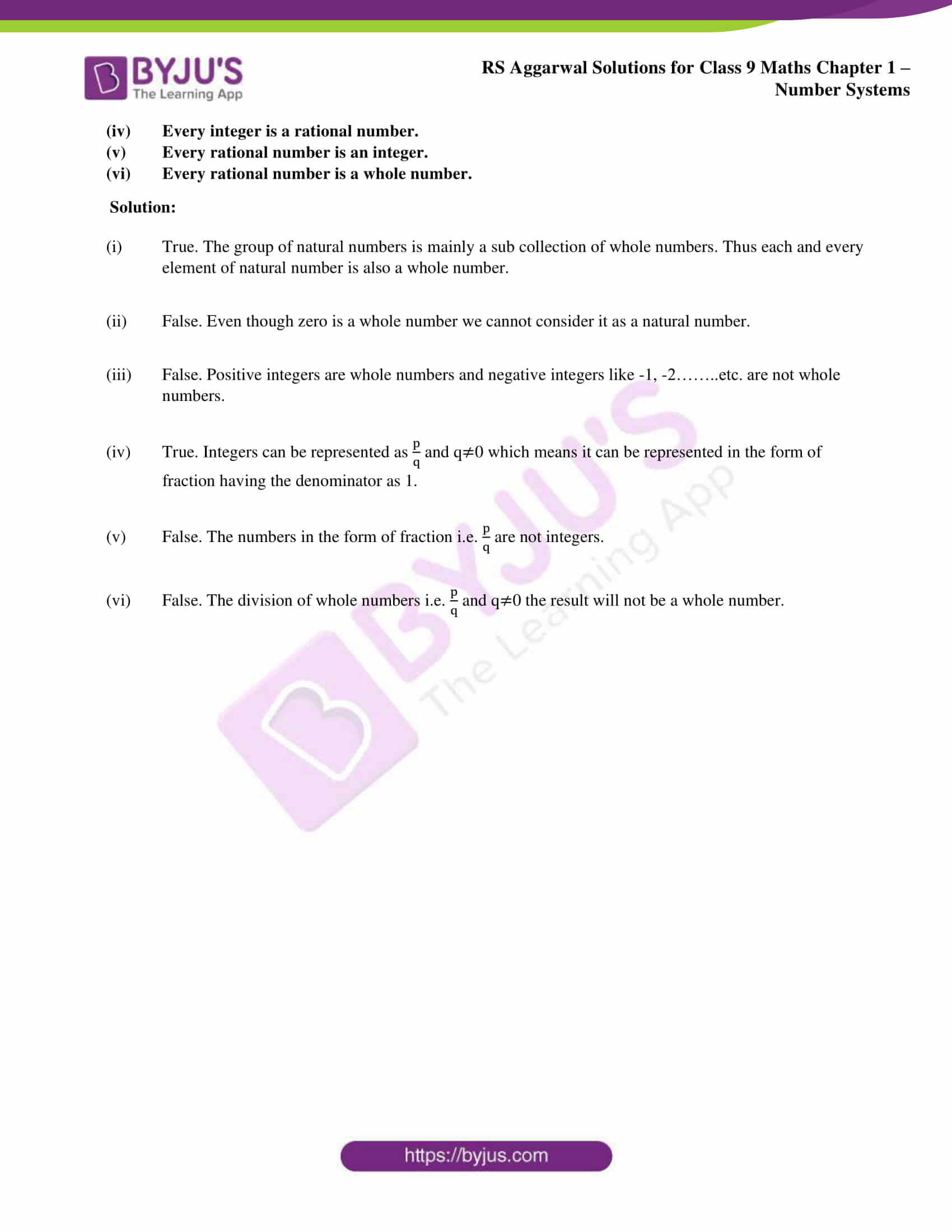### Access other exercise solutions of class 9 Maths Chapter 1: Number Systems

Exercise 1B Solutions 5 Questions

Exercise 1C Solutions 14 Questions

Exercise 1D Solutions 8 Questions

Exercise 1E Solutions 10 Questions

Exercise 1F Solutions 25 Questions

Exercise 1G Solutions 18 Questions

### RS Aggarwal Solutions Class 9 Maths Chapter 1 – Number Systems Exercise 1A

RS Aggarwal Solutions Class 9 Maths Chapter 1 Number Systems Exercise 1A contains solutions for problems to find rational numbers between two numbers using different methods.

### Key features of RS Aggarwal Solutions for Class 9 Maths Chapter 1: Number Systems Exercise 1A

• Students can use the PDF as a reference material according to RS Aggarwal Solutions for Class 9 to solve exercise 1A.
• The step by step solutions prepared by our team of expert faculties help students to improve their confidence to solve the problems and appear for the exams without fear.
• The solutions prepared are according to the RS Aggarwal Solutions guidelines to understand the evaluation used for each step.
• Many number of questions are added in each exercise to help students study for the exams accordingly.